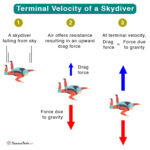Home / Physics / Can Cats Survive Terminal Velocity?

# Can Cats Survive Terminal Velocity?

Cats have a unique tendency to survive a fall. When a cat jumps or slips from a tall building and lands on flat ground, it can absorb the impact of landing. The reason is that a cat’s terminal velocity is sufficiently low and much less than a human’s. The following equation gives the terminal velocity.

$v_{T} = \sqrt{\frac{2mg}{C\rho_{air} A}}$

Let us calculate the terminal velocity of a cat using the following values.

m = 5 kg

ρair = 1.29 kg/m3

C = 1.1

A = 0.1 m2

g = 9.81 m/s2

Plugging them in, we get

$v_T = \sqrt{\frac{2 \cdot 5 \hspace{1mm} kg \cdot 9.81\hspace{1mm} m/s^2}{1.1 \cdot 1.29\hspace{1mm} kg/m^3 \cdot 0.1\hspace{1mm} m^2}}\\ \Rightarrow v_T = 26.3 \hspace{1mm} m/s \hspace{1mm} \text{or} \hspace{1mm} 59 \hspace{1mm} mph$

Thus, a cat has a terminal velocity of approximately 60 mph.

A cat can sense when she is accelerating, and her instincts kick in. She will rotate and let herself to a position to brace for landing. Past studies have shown that a cat falling from a 32 stories tall building will achieve terminal velocity until she has fallen through a height equivalent to five stories. During the fall, she relaxes by splaying her legs and taking the spread-eagle stance. This position gives her a large surface area, brings her to terminal velocity, and allows her to absorb the impact.

Article was last reviewed on Wednesday, June 22, 2022

### Related articlesWhat is the Terminal Velocity of a Human?Types of Energy